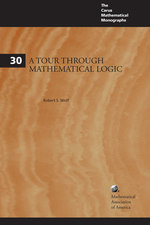Have library access? Log in through your library# A Tour through Mathematical Logic

Robert S. Wolf
Volume: 30
Copyright Date: 2005
Edition: 1
Pages: 414
https://www.jstor.org/stable/10.4169/j.ctt5hh94h

## Table of Contents

1. Front Matter
(pp. i-vi)
2. Preface
(pp. vii-xii)
3. Table of Contents
(pp. xiii-xvi)
4. CHAPTER 1 Predicate Logic
(pp. 1-58)

Why should students of mathematics want to know something about predicate logic? Here is one answer: predicate logic helps one understand the fine points of mathematical language, including the ambiguities that arise from the use ofnaturallanguage (English, French, etc.) in mathematics. For research mathematicians, these ambiguities are usually easy to spot, and their intuition will normally prevent any errors. Yet, occasionally, such ambiguities can be very subtle and present real pitfalls. For instructors of mathematics, awareness of this type of ambiguity is indispensable for analyzing students’ mistakes and misconceptions.

Let’s illustrate this point with a rather fanciful example:...

5. CHAPTER 2 Axiomatic Set Theory
(pp. 59-94)

Why should students of mathematics want to know something about axiomatic set theory? Here is one answer: set theory provides a natural and efficient framework in which all of contemporary mathematics can be unified. We live in a time when the specialization within mathematics (and many other disciplines, of course) is mind-boggling, and even though that specialization is a tribute to the great success of mathematics and mathematicians, it can be alienating. Not too many centuries ago, the few professional mathematicians generally understood just about all of the mathematics of their day, and many of them also did important work...

6. CHAPTER 3 Recursion Theory and Computability
(pp. 95-134)

Why should students of mathematics want to know something about recursion theory and computability? Here is one answer that applies to many other people beside mathematicians: a rudimentary knowledge of these subjects helps one to understand what tasks can and can not be done by digital computers. To be sure, today’s powerful computers, with their slick graphical interfaces and “continuous” input and output devices (the mouse, speech, etc.), are very different from the simple computing devices such as Turing machines that are studied in elementary recursion theory. Yet, in an important sense, the most powerful supercomputer is still equivalent to...

7. CHAPTER 4 Gödel’s Incompleteness Theorems
(pp. 135-164)

Why should students of mathematics want to know something about Gödel’s incompleteness theorems? Here is one answer: as we mentioned in Chapter 3, they are possibly the most philosophically significant results in the history of mathematics. These theorems expose substantial and unavoidable limitations on the power and perhaps even the reliability of mathematics. They are remarkably similar in flavor to the uncertainty principle in physics, another amazing discovery of the early part of the twentieth century. The undecidable statements in Gödel’s results are unnatural and contrived, but in the last section of this chapter we will discuss some more recent...

8. CHAPTER 5 Model Theory
(pp. 165-224)

Why should students of mathematics want to know something about model theory? Here is one answer: model theory helps one to understand what it takes to specify a mathematical structure uniquely.

Let us consider what is undoubtedly the most well-known problem of this sort, the problem of defining or characterizing the field of real numbers. (These are really two separate problems; we are more concerned with characterization.) This was a major concern in the eighteenth and nineteenth centuries. One of the main reasons it took nearly two centuries after the invention of calculus to put the subject on a firm...

9. CHAPTER 6 Contemporary Set Theory
(pp. 225-278)

Why should students of mathematics want to know something about contemporary set theory? Here is one answer: the independence results that sparked the modern era of set theory are surprising and fascinating, in some ways even more so than the incompleteness theorems discussed in Chapter 4. That’s because the statements that were shown to be independent of set theory in the 1960s, notably the axiom of choice and the continuum hypothesis, are basic and natural mathematical statements that many people thought should be settled by a sensible set of axioms for set theory such as ZF. In contrast, none of...

10. CHAPTER 7 Nonstandard Analysis
(pp. 279-316)

Why should students of mathematics want to know something about nonstandard analysis? Here is one answer: nonstandard analysis provides a powerful tool for understanding and using the main ideas of calculus, analysis, and other branches of mathematics. This tool can be very helpful to students and teachers of mathematics as well as those doing mathematical research.

Contrary to what many mathematicians may believe, nonstandard analysis is much more than a clever, unorthodox approach to calculus and real analysis. Nonstandard methods have been useful in almost every branch of mathematics and even subjects outside of mathematics. For example, the anthology [Cut]...

11. CHAPTER 8 Constructive Mathematics
(pp. 317-346)

Why should students of mathematics want to know something about constructive mathematics? Here is one answer: the constructivist school of mathematics shows us that there is a whole spectrum of mathematical activity, from arithmetical operations and other purely computational processes at one end, to abstract reasoning about very “remote” objects at the other end. Being aware of this spectrum, and understanding how to identify the “constructive” (or “computational” or “numerical”) content of a given argument or definition, can make one a better mathematician.

Here is another, rather simple answer to this question: computers are becoming more and more important as...

12. APPENDIX A A Deductive System for First-order Logic
(pp. 347-348)
13. APPENDIX B Relations and Orderings
(pp. 349-354)
14. APPENDIX C Cardinal Arithmetic
(pp. 355-360)
15. APPENDIX D Groups, Rings, and Fields
(pp. 361-374)
16. Bibliography
(pp. 375-380)
17. Symbols and Notation
(pp. 381-386)
18. Index
(pp. 387-397)